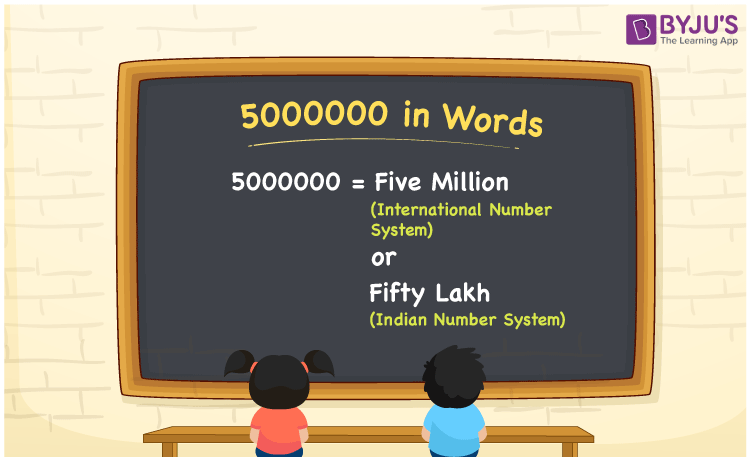# 5000000 in Words

We can write 5000000 in words as Five million in the International number system and as Fifty lakh in the Indian number system. In this article, you will learn how to convert the cardinal number 5000000 to words of both International and Indian number systems.

 5000000 in words Five million (or) Fifty lakh Five million  (or) Fifty lakh in Numbers 5000000

## 5000000 in English Words

We generally write numbers in words using the English alphabet. Thus, we can read 5000000 in English as “Five million” or “Fifty lakh”.## How to Write 5000000 in Words?

5000000 in words – International number system

The below table depicts the place value chart for 5000000 with respect to the International number system.

 Millions Hundred thousands Ten thousands Thousands Hundreds Tens Ones 5 0 0 0 0 0 0

Here, ones = 0, tens = 0, hundreds = 0, thousands = 0, Ten thousands = 0, Hundred thousands = 0, millions = 5

Therefore, 5000000 in words = 5 million = Five million

5000000 in words – Indian number system

Given below is the place value chart for 5000000 in the Indian system.

 Ten lakhs Lakhs Ten thousands Thousands Hundreds Tens Ones 5 0 0 0 0 0 0

Here, ones = 0, tens = 0, hundreds = 0, thousands = 0, ten thousands = 0, lakhs = 0, ten lakhs = 5

That means, 50 lakhs

= Fifty lakhs

5000000 is a natural number that precedes 5000001 and succeeds 4999999.

5000000 in words – Five million (or) Fifty lakhs

Is 5000000 an odd number? – No

Is 5000000 an even number? – Yes

Is 5000000 a perfect square number? – No

Is 5000000 a perfect cube number? – No

Is 5000000 a prime number? – No

Is 5000000 a composite number? – Yes

## Frequently Asked Questions on 5000000 in Words

Q1

### How do you say 5000000 in words?

We can say 5000000 in words as Five million (or) Fifty lakh.
Q2

### How do you write Rs. 5000000 in words for a cheque?

For a cheque, we write Rs. 5000000 in words as “Fifty lakh rupees only”.
Q3

### Is 5000000 the composite number?

Yes, 5000000 is a composite number